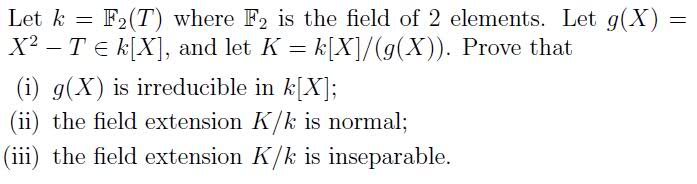# Irreducibility and finite fieldsTrying to do i) and iii) on this past exam paper

For part i) I'm pretty stumped

I've said that the possible roots of the polynomial are +- all the factors of T

In particular rt(T) needs to be a factor of T but this can't be possible?

Doesn't sound too good but its the best I've got.

Part iii) I know this means that every element in K is seperable over k, i.e that the minimal polynomials of elements in K are seperable, where they have no repeated roots.

Not sure how to apply this though..

Maybe K = k(rt(T))

so the minimal polynomial of K is X^2 - T which has repeated root rt(T) so it is inseperable?

Last edited:

For part i:

[STRIKE]Because this is a finite field, you must show that the polynomial g(X) has no roots in k. Because k is a finite field of 2 elements, you can just try plugging in 0 and 1 because k = {0,1}.

So g(X) is irreducible if and only if 0^2-T is not equal to zero and 1^2-T is not equal to zero. Since T is an extended element onto k and is therefore not equal to either 0 or 1, which is what we need to make the above two equations equal to zero, I think that we can safely draw our conclusion. What do you think?[/STRIKE]

edit: i'm dumb. i'll rethink this.

For part i:

Because this is a finite field, you must show that the polynomial g(X) has no roots in k. Because k is a finite field of 2 elements, you can just try plugging in 0 and 1 because k = {0,1}.

So g(X) is irreducible if and only if 0^2-T is not equal to zero and 1^2-T is not equal to zero. Since T is an extended element onto k, I think we can safely draw our conclusion. What do you think?

isn't k = {0, 1, T, 1+T} since k = {a + bT | a, b in {0,1}}

Otherwise I see what you mean. Thanks for the reply

yeah you're completely right and i noticed my mistake right after i posted that. i overlooked the whole extended element thing. my bad.

bump for confirmation